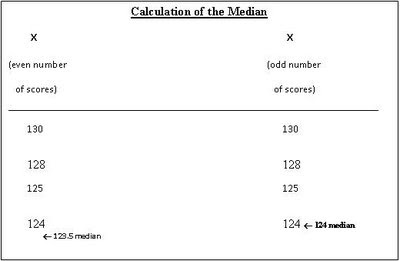## Friday, March 13, 2009

### Understanding the Numbers-IV

It's been awhile since we focused on statistics and test interpretation so I thought we could talk about the measures of central tendency (i.e. mean, median, and mode). Again, most of the information that you will read on this topic are from Jerome Sattler's "Measurement of Children."

Measures of Central Tendency

The three most commonly used measures of central tendency, the typical or representative score, are the mean, median and mode.

Mean
The mean is the arithmetic average of all the scores in a set of scores. To obtain the mean, divide the sum of all the scores by the total number of scores in the set (N). The formula is as follows:The mean is responsive to the exact position of each score in the distribution, but it is also sensitive to a few relatively extreme scores. Consequently, extreme scores in a set can distort the mean. Overall, the mean is the preferred measure of central tendency. It is appropriate for both interval and ratio scale data.

Median
The median is defined as the middle point in a set of scores arranged in order of magnitude. Fifty percent of the scores lie at or above the median and fifty percent of the scores lie at or below the median. If there are an even number of scores, the median is the number halfway between the two middlemost scores and therefore is not any of the actual scores. If there are an odd number of scores, the median is simply the middlemost score.

To calculate the median, arrange the scores in order of magnitude from highest to lowest. Then count up (or down) halfway through the scores (see table below).When distributions are “skewed” (the bulk of the scores are at either the high or the low end of the set), the median is a better measure than the mean of the typical point in the set. The median is less affected by outliers, scores that deviate extremely from the other scores in the set. The median is an appropriate measure of central tendency for ordinal, interval, or ratio scale data. The median is the best measure of central tendency when distributions are “skewed.”

Mode
The mode of a set of scores is the score that occurs more often than any other. In some sets two scores occur more often than any other score and with the same frequency; in such cases the distribution is said to be bimodal. When more than two scores occur more frequently than any other score and with the same frequency, the distribution is said to be multimodal.

The mode is greatly affected by chance and has little or no mathematical usefulness. However, it does tell us what score is most likely to occur and hence is useful in analyzing qualitative data 9for example, “What was the most frequently occurring classification in the group?”). It is the only appropriate measure of central tendency for nominal scale data.

MDZE3SGDZH9Y